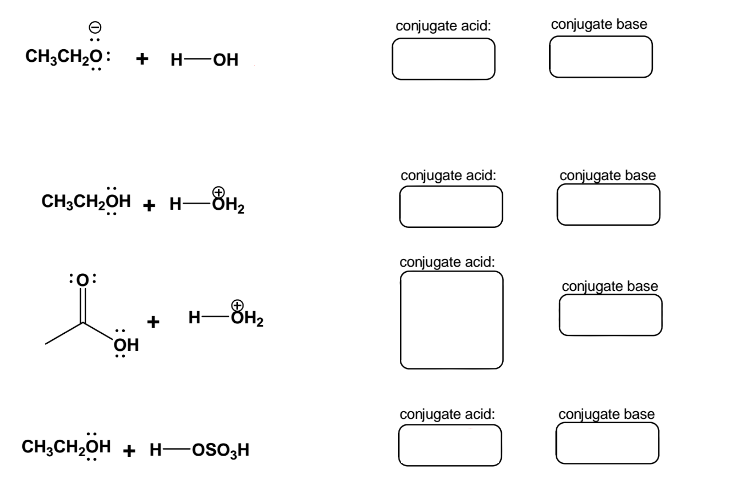Problem: Write the products for the following acid-base reaction. a) These reactions will be in equilibrium, and the equilibrium can favor one side or the other or be balanced (i.e. nearly equal). Indicate which side of the reaction is favored in the equilibrium either by use of arrows ⇌ or writing on one side of the reaction, “favored”. b) Draw the curved arrows to show the flow of e - for each of the reaction.

FREE Expert Solution
97% (356 ratings)
Problem Details

Write the products for the following acid-base reaction.

a) These reactions will be in equilibrium, and the equilibrium can favor one side or the other or be balanced (i.e. nearly equal). Indicate which side of the reaction is favored in the equilibrium either by use of arrows ⇌ or writing on one side of the reaction, “favored”.

b) Draw the curved arrows to show the flow of e for each of the reaction.What scientific concept do you need to know in order to solve this problem?

Our tutors have indicated that to solve this problem you will need to apply the Acid Base Equilibrium concept. You can view video lessons to learn Acid Base Equilibrium Or if you need more Acid Base Equilibrium practice, you can also practice Acid Base Equilibrium practice problems .

What is the difficulty of this problem?

Our tutors rated the difficulty of Write the products for the following acid-base reaction. a)... as medium difficulty.

How long does this problem take to solve?

Our expert Organic tutor, Jonathan took 8 minutes to solve this problem. You can follow their steps in the video explanation above.

What professor is this problem relevant for?

Based on our data, we think this problem is relevant for Professor Periche's class at LEHMAN-CUNY.